Want to share your content on R-bloggers? click here if you have a blog, or here if you don't.Previously I posted on how to apply gradient descent on linear regression as an example. With that as background it’s relatively easy to extend the logic to other problems. One of those is matrix factorisation.

There are many ways to factorise a matrix into components such as PCA, singular value decomposition (SVD), but one way is to use gradient descent. While it’s inception is in image processing, it was popularised by it’s use with recommender systems (Funk SVD). But, it is also found useful in other ways such as market basket analysis and topic modelling. Once you know the nuts and bolts and how to apply it you’ll find uses that are not recommenders.

## Matrix factorisation

Firstly, the maths.

Consider a matrix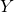. We want to factoriseinto two matrices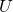andsuch that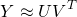andhave dimensions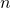x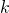and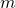xwhere typically. These are estimated in the following steps 1) initialise each matrix with random values 2) iteratively adjust each matrix by the direction of negative gradient to minimise the MSE and 3) stop at the maximum iterations or a specified tolerance.

The objective function is the mean squared error and a regularisation term.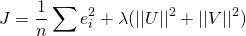Here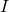is an indicator whereif the rating or value exists and 0 if it is missing. The gradients with respect toandare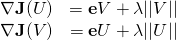The factor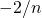is dropped to keep it tidy. By doing so this quantity is effectively rolled into the learning rate, if you work through the maths. For each iterationandare adjusted by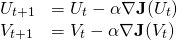whereis the learning rate.

## Example

Factorising a matrix into two lower dimensional matrices learns the latent information. For example, if your data is a patient-drug profile it may learn the diagnosis of the patient. In the case of a recommender if your data are song ratings it will learn those that prefer metal over pop, classical over electro, etc. The first column of thematrix may represent the level of distortion in a song, or tempo as a numeric value. What the algorithm picks out as important isn’t necessarily obvious and worth inspecting those on the extremes to get a feel for the data. The user matrixworks similarly. The numeric values in the user row represent how much weight that user gives to the particular feature.

Why this is useful over PCA or SVD is it handles missing values. The other methods treat every cell as a value so need to be imputed before factorising. It will then use the imputed values to learn the data structure. The beauty of this method is it factorises only on the observed values and imputes the missings.

I’ve developed a simple package to apply the method. There are other packages which are more optimised but this is just simple and easy to use. You can install it from Github (not on CRAN).

devtools::install_github("doehm/matrixfactorisation")

Simulate some data.

m <- matrix(sample(c(NA, 1:5), 60, replace = TRUE, prob = c(0.2, rep(0.8/5, 5))), nrow = 10)
m

##       [,1] [,2] [,3] [,4] [,5] [,6]
##  [1,]   NA   NA    1    4    2    2
##  [2,]   NA    2   NA    1    5    4
##  [3,]    5    3    3    1    4    4
##  [4,]   NA    1    3    5    3    5
##  [5,]    4    4    3    2    4    3
##  [6,]   NA    4    5   NA    4    4
##  [7,]   NA   NA    2    3    3    2
##  [8,]    2   NA    2   NA    3    2
##  [9,]    2    3    5    2    3   NA
## [10,]    3    3    2    2    5    5

Select a test set and factorise. Selecting a test set holds out a proportion of randomly selected observations. It ensures there is at least 1 observation remaining in each row. This can be increased by min_row.

id <- select_test(m, 0.2)
mf <- matrix_factorisation(m, 2, test = id$test, pbar = TRUE) ## 00:00:02 // dimensions 2 // epoch 1000 // train 1.2703 // test 1.6059 // delta 9.467e-04 Plot the convergence. plot(mf)Viewing the convergence profile is useful to see whether or not the data has been overfit. ## Clustering data with missing values Typically missing values are treated before clustering which may include removing certain rows or columns. Usually this also means throwing away actual observations. If the data has a proportionally large amount of missing values you could be throwing away most of the data. This is quite typical for ‘user-item’ type data. Matrix factorisation enables the use of more data. This is demonstrated in a small example. A matrix is randomised with 12 users, 6 items and 2 groups with a high degree of missingness. The group is the latent variable. The values are drawn from a Poisson distribution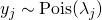. Those that are in group 1 are drawn from the distribution with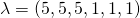and those in group 2 are drawn from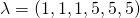. It is then simple to see the relationship between the users, items and groups. Count data is very common and often has it’s own challenges when clustering, particularly when counts are small or only part of the units history is observed e.g. a data set where the grain is the patient, the items are prescription drugs and the values are counts of that drug. user_a <- function(m) c(rpois(m/2, 5), rpois(m/2, 1)) user_b <- function(m) c(rpois(m/2, 1), rpois(m/2, 5)) df <- NULL n <- 12 m <- 6 grp <- as.factor(sort(rep(letters[1:2], n/2))) for(k in 1:n){ df <- rbind(df, if(k <= 6) user_a(m) else user_b(m)) } df ## [,1] [,2] [,3] [,4] [,5] [,6] ## [1,] 6 7 6 0 3 2 ## [2,] 5 5 3 1 1 1 ## [3,] 7 3 7 1 0 0 ## [4,] 5 5 5 0 0 0 ## [5,] 3 1 3 2 0 0 ## [6,] 5 7 4 0 1 0 ## [7,] 0 0 2 7 6 6 ## [8,] 3 1 0 6 9 8 ## [9,] 2 0 2 6 4 5 ## [10,] 0 2 1 5 4 9 ## [11,] 0 2 2 4 4 5 ## [12,] 0 0 2 1 6 8 We’ll now suppress 60% of the values. df_na <- select_test(df, 0.6)$train
df_na

##       [,1] [,2] [,3] [,4] [,5] [,6]
##  [1,]   NA    7   NA   NA   NA    2
##  [2,]    5   NA    3   NA   NA   NA
##  [3,]   NA    3    7   NA    0   NA
##  [4,]    5    5    5    0    0   NA
##  [5,]    3    1    3   NA   NA    0
##  [6,]    5   NA   NA    0   NA    0
##  [7,]    0   NA   NA   NA   NA    6
##  [8,]    3    1   NA    6    9    8
##  [9,]   NA    0    2   NA    4   NA
## [10,]    0    2   NA   NA   NA    9
## [11,]    0    2    2   NA   NA    5
## [12,]   NA   NA    2   NA    6   NA

This data can be challenging to use, but by factorising using only the observed data it is embedded in a much more usable form (mathematically this is similar to using the autoencoder – PCA vs autoencoders). Using the prediction matrix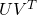all units can be clustered. While there is error in the prediction matrix it should be accurate enough to find the two groups.

# factorise
df_mf <- matrix_factorisation(df_na, 2, epochs = 2000)

# cluster
km <- kmeans(df_mf$pred, 2, nstart = 100) clus <- as.factor(km$cluster)
names(clus) <- grp
clus

## a a a a a a b b b b b b
## 1 1 1 1 1 1 2 2 2 2 2 2
## Levels: 1 2

table(clus, grp)

##     grp
## clus a b
##    1 6 0
##    2 0 6

A simple K-means clustering process finds the two groups, which is encouraging with a highly patchy and small data set. K-means can struggle with low value count data so embedding the data in this form can really help.

Often the original data set can be large and therefore inefficient to cluster. The same results (or a at least a very good approximation) is achieved using thematrix instead of the prediction matrix.

# cluster
km <- kmeans(df_mf$u, 2, nstart = 100) clus <- as.factor(km$cluster)
names(clus) <- grp
clus

## a a a a a a b b b b b b
## 1 1 1 1 1 1 2 2 2 2 2 2
## Levels: 1 2

table(clus, grp)

##     grp
## clus a b
##    1 6 0
##    2 0 6

In this case we achieve the same result which is great. This is an approximation so may not always work as well, in fact if you randomised the input matrix a number of times I’m sure you’ll see different results. But in general you should achieve very reasonable results most of the time.

In practice I have had success using this technique on large data sets. While my preferred method is to use the autoencoder for dimension reduction and embedding, there is an overhead in setting it up. On the other hand matrix factorisation is quick and simple to get you exploring the data sooner. Clustering is just one example and I’m sure you’ll find more applications that benefit from matrix factorisation.

The post Use more of your data with matrix factorisation appeared first on Daniel Oehm | Gradient Descending.